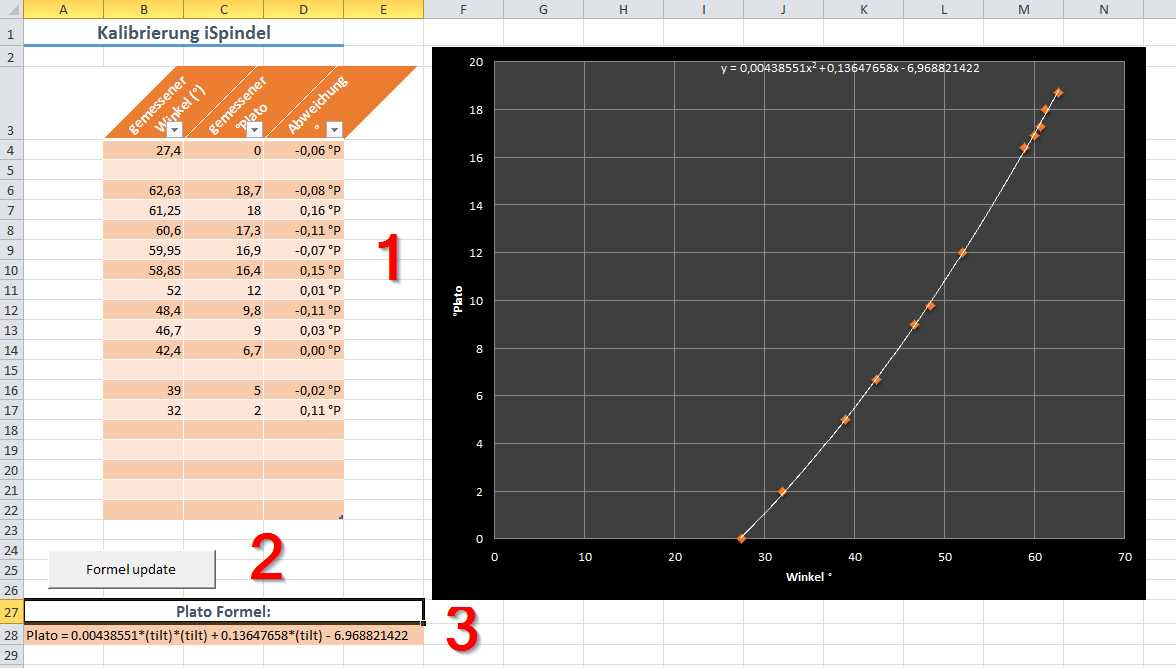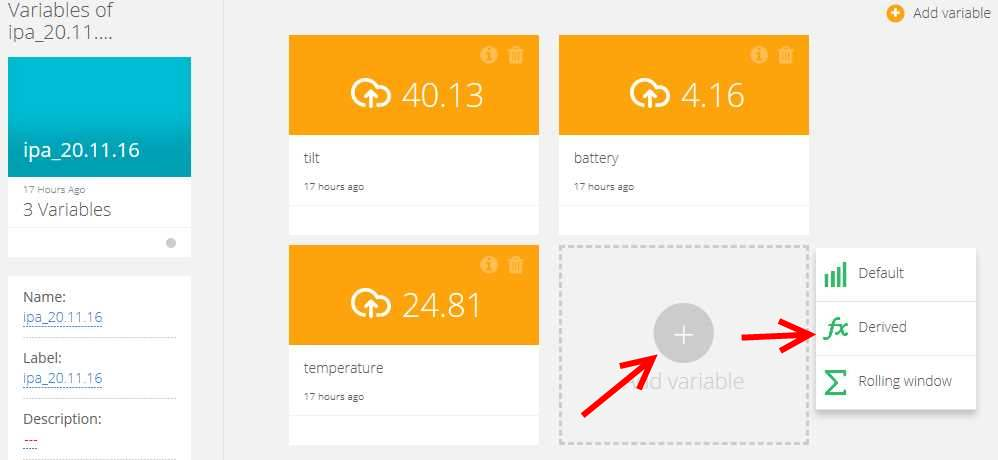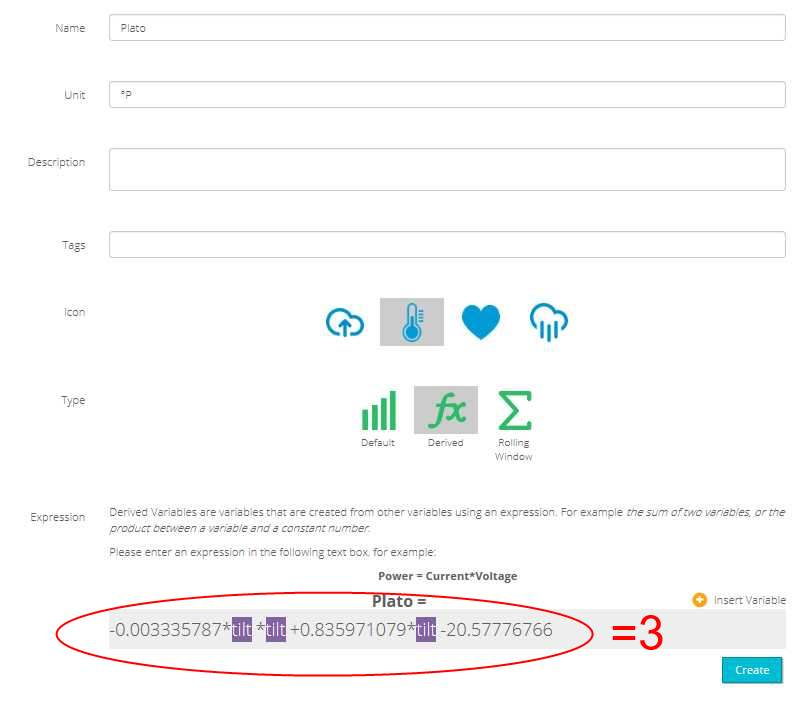Calibration

The tilt of the iSpindle floating in the wort can be converted into degrees Plato (ºPlato), density (SG) or other units. To be able to do so, a reference should be made between the degrees of tilt of the iSpindle and the chosen unit. Reference values will have to be measured and with the use of an Excel table a graph and formula is made. Excel will calculate the relation between the tilt of the iSpindle and Plato degrees, density or others. As each self built iSpindle will differ, the reference measurement is needed to be done for each individual iSpindle, but only once.

Content

Easy method (I)

It’s recommended you connect the iSpindel with Ubidots for easy reading of the measured tilts. Furthermore it is wise to change settings of the iSpindel so it sends new readings every 20 seconds. With such a short interval the measurements can be easily followed on the Ubidots website.

1. Put the iSpindel in clean water (0 degrees Plato, SG 1.000).

Write down the tilt of the iSpindel

2. Make a solution of sugar in water with the highest anticipated density. If you are brewing strong beers with a high starting density, you should have reference measurements in this high range. For most brewers a solution with a density around 1.085 or around 20 degrees Plato will do.

Example for sugar solution: 400 ml with 100 g sugar
Measure the density or the degrees Plato and write it down with the tilt of the iSpindel

3. Dilute the solution above with water until it reaches 15 degrees Plato or density 1.061

Example for sugar solution: dilute the solution of point 2 with 166 ml of water
Measure the density or the degrees Plato and write it down with the tilt of the iSpindel

4. Dilute the solution from the point above till it reaches approximately 10 degrees Plato, SG 1.040 of 10 degrees Brix.

Example: Dilute the solution from point 3 with 333 ml of water.
Measure the density or the degrees Plato and write it down with the tilt of the iSpindel

5. Dilute the solution from the point above till it reaches approximately 7.5 degrees Plato, SG 1.030 or 7.5 degrees Brix.

Example: Dilute the solution from point 4 with 333 ml of water.
Measure the density or the degrees Plato and write it down with the tilt of the iSpindel

6. Dilute the solution from the point above till it reaches approximately 5 degrees Plato, SG 1.020 or 5 degrees Brix

Example: Dilute the solution from point 5 with 666 ml of water
Measure the density or the degrees Plato and write it down with the tilt of the iSpindel

7. Dilute the solution from the point above till it reaches approximately 2.5 degrees Plato, SG 1.010 or 2.5 degrees Brix.

Example: Dilute the solution from point 6 with 2000 ml of water.
Measure the density or the degrees Plato and write it down with the tilt of the iSpindel
Enter the measured points in the Excel sheet (see below). This Excel sheet will calculate the formula for the reference

Improved method using fast fermenting (II)

This method is more precise since it will take the affect of CO2 formed during fermenting into account.

It’s recommended you connect the iSpindel with Ubidots for easy reading of the measured tilts. Furthermore it is wise to change settings of the iSpindel so it sends new readings every 20 seconds. With such a short interval the measurements can be easily followed on the Ubidots website.

1. Put the iSpindel in clean (tap) water (0 º Plato or SG 1.000)

Write down the measured tilt of the iSpindel

2. Make a solution of sugar in water with the highest anticipated density. If you are brewing strong beers with a high starting density you should have reference measurements in this high range. For most brewers a solution with a density around 1.085 or around 20 degrees Plato will do.

Example: 800 ml of water + 200 g crystal sugar.

3. Add yeast to the sugar solution and let the iSpindel float in the solution

Measure the density or the degrees Plato and write it down with the tilt of the iSpindel

4. Measure the density of the fluid and the corresponding tilt of the iSpindle at regular intervals till the end of fermentation has been reached.

Enter the measured values in the Excel sheet. This sheet will show the formula of the calibration curve.

**Caution: if you measure in Brix value with a refractometer you have to recalculate the measured value to correct for the alcohol reading*

Formula

• Online Gravity Calibration tool

The measured values can be entered in the field SG/Plato or BRIX plus the corresponding Tilt. It will calculate a formula of 2nd or 3rd degree that will predict the density or the degrees of Plato given an tilt value. This formula has to be entered in Firmware to be able to get readings in density or degrees Plato.

• Excel Sheet

The measured values can be entered in the Excel sheet. This sheet will calculate a formula that will predict the density or the degrees of Plato given an tilt value. This formula has to be entered in Firmware to be able to get readings in density or degrees Plato. Other readings, such as apparent attenuation or alcohol % can be obtained in similar manners with the use of a ‘Derived Variable’ in Ubidots.

1. First enter the measured values in Excel: